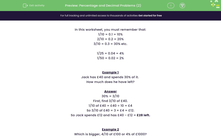# Percentage and Decimal Problems (2)

In this worksheet, students solve problems which require knowing the equivalents of those fractions with a denominator of a multiple of 10 or 25.Key stage:  KS 2

Curriculum topic:   Number: Fractions, Decimals and Percentages

Curriculum subtopic:   Solve Percentage/Decimal Problems

Difficulty level:#### Worksheet Overview

In this worksheet, you must remember that:

1/10 = 0.1 = 10%

2/10 = 0.2 = 20%

3/10 = 0.3 = 30% etc.

1/25 = 0.04 = 4%

1/50 = 0.02 = 2%

Example 1

Jack has £40 and spends 30% of it.

How much does he have left?

30% = 3/10

First, find 3/10 of £40.

1/10 of £40 = £40 ÷ 10 = £4

So 3/10 of £40 = 3 × £4 = £12.

So Jack spends £12 and has £40 - £12 = £28 left.

Example 2

Which is bigger, 4/10 of £100 or 4% of £1000?

1/10 of £100 = £100 ÷ 10 = £10

4/10 of £100 = 4 × £10 = £40

4% = 1/25

1/25 of £1000 = £1000 ÷ 25 = £40

They are the same.

### What is EdPlace?

We're your National Curriculum aligned online education content provider helping each child succeed in English, maths and science from year 1 to GCSE. With an EdPlace account you’ll be able to track and measure progress, helping each child achieve their best. We build confidence and attainment by personalising each child’s learning at a level that suits them.

Get started